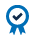A basis point is a unit of measure used in finance to describe the percentage change in the value or rate of a financial instrument.

One basis point is equivalent to 0.01% (1/100 of a percent) or 0.0001 in decimal form. If interest rates rose from 2.00% to 2.50%, it would be said that rates rose 50 basis points. In many cases, basis point refers to changes in short-term interest rates, such as Eurodollars, but it is also important with longer-term bond yields.

Basis Point Value, also known as DV01 (the dollar value of a one basis point move) represents the change in the value of an asset due to a 0.01% change in the yield.

BPV or DV01 calculations are used in many ways, but primarily to show the dollar amount of change for each increase or decrease in interest rates. If the value of the Eurodollar futures contract moves by one basis point (.01%), it would equate into a \$25.00 move in the contract value. If Eurodollar futures moved four basis points or .04%, it would equate to a \$100 move in the value of the contract.

Show graphic calculating this BPV or DV01  for Eurodollars:

## Basis Point Value Calculation

The face value of the Eurodollar futures contract is \$ 1,000,000. The futures track three-month Eurodollar rates (three-month ICE LIBOR) hence we use 90 days in the equation, and .01% in decimal form is .0001.

Basis Point Value (BPV)  =  Face Value x (#days ÷ 360) x .01%

BPV = 1,000,000 x (90 ÷ 360) x .0001

BPV = \$25.00

### Example

This example shows Eurodollars in terms of the IMM Price index. Assume Eurodollar interest rates rose from 1.00% to 1.05%, this would represent a .05% or five basis point rise in Eurodollar interest rates. But remember from the prior modules that Eurodollar futures are priced off the IMM price index.

IMM price index = 100 – Eurodollar rate (or three month ICE LIBOR)

In the example above, Eurodollars were at 1.00%. The IMM price index, therefore, would be 100 – 1.00 = 99.00. Subsequently, interest rates rose to 1.05%. The IMM price index at that point would be 100 – 1.05   = 98.95.

As you can see, interest rate prices move inversely with interest rate yields. As rates rose five basis points, the Eurodollar IMM price index declined from 99.00 to 98.95.

To find out how much that means in terms of dollar value, we have to convert basis point movement into dollar movement. This requires knowing the DV01 (dollar Value of a .01 move)

The basis point value in Eurodollar futures from our calculation above is \$25.00.  Therefore, a five basis point move equates to \$125.00

5 basis points x \$25.00/basis point = \$125.00.

## Basis Points and Tick Size in Eurodollar Futures

The minimum allowable price fluctuation, or tick size, is generally established at ½ basis point., or .005%. Based on a million-dollar face value 90-day instrument, this equates to \$12.50. However, in the nearby expiring contract month, the minimum price fluctuation is set at 1/4 basis point, or .0025%, equating to \$6.25 per contract.

### Nearby Expiring Contract:

One Tick (.0025 basis pts) = \$6.25

Tick Movement Quote
Starting price 99.0000
Increased one tick (.0025 basis points) 99.0025
Increased two ticks (.0050 basis points) 99.0050
Increased three ticks (.0075 basis points) 99.0075

### All Other Expiring Contracts:

One Tick (.005 basis pts) = \$12.50

Tick Movement Quote
Starting price 99.000
Increased one tick (.005 basis points) 99.005
Increased two ticks (.010 basis points) 99.010
Increased three ticks (.015 basis points) 99.015

#### ACCREDITED COURSEDid you know that CME Institute classes can fulfill CFA and GARP continuing education requirements? Every CME Institute course can be self-reported in your CFA online CE tracker and select classes can be used for GARP credits. See which of our classes qualify for GARP credits here.